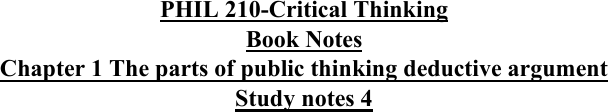# PHIL 210 Study Guide - Quiz Guide: Deductive Reasoning, Natural Number, Modus Ponens

7 views3 pagesPHIL 210-Critical Thinking
Book Notes
Chapter 1 The parts of public thinking deductive argument
Study notes 4
Arguments that is Invalid and Not Sound
o 1. 42 is number.
o 2. Continents Drift.
o 3. Pigs grunt.
Therefore,
o 4. Socrates is Greek
. P1, P2, and P3 are all true, the conclusion is true as well. But the premises do not relate
to nor do they support the conclusion, therefore it is not valid, therefore not sound.
Argument that is Sound
o 1. Vixens are foxes.
o 2. All foxes are mammals.
Therefore
o 3. Vixens are mammals.
The premises relate to and supports the conclusion in a way that is premises are true
conclusion is true, therefore is it Valid, All the premises are true.
o This argument is sound. This is also an example of an argument framed in
categorical terms: manner of how the categories vixen, fox, and mammal are
related.
Conditional Reasoning: ( If P then Q )
Quantifier Expression: ( like “some” and “every”) the previous example
Variable: ( like x or y kind of place holder for objects that could be plugged into the
statement)
o Ex:
1. For every object x, if x is a vixen then x is a fox.
2. For every object x, if x is a fox then x is a mammal.
Therefore
3. For every object x, if x is a vixen then x is a mammal
Hypothetical Syllogism
o 1. If P, then Q.
o 2. If Q, then Z.
Therefore,
o 3. If P then Z.
Disjunctive Syllogism
o 1. P or Q.
o 2. Not Q.
Therefore,
Unlock document

This preview shows page 1 of the document.
Unlock all 3 pages and 3 million more documents.

Already have an account? Log in

# Get access

Grade+
\$10 USD/m
Billed \$120 USD annually
Homework Help
Class Notes
Textbook Notes
40 Verified Answers
Study Guides
1 Booster Class